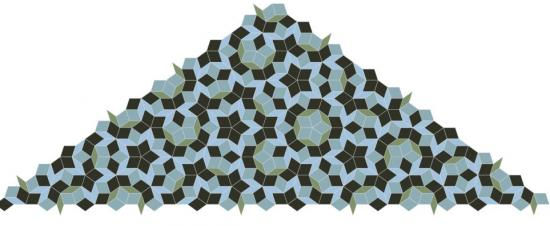# Penrose Tiling

Posted by Mark M on Feb 23, 2008This is a short script to generate the non-periodic penrose tiling. As mentioned under the L-system posting a while back this can be produced by means of a well-known l-system recipe. The L-system works beautifully but it is very difficult to see how it works. This script uses a contraction method of starting with one half of one of the tiles and recursively breaking it down into smaller units. One of the goals was to show the intense relationship between the tiling and the golden ratio and also to see if it could be done using nothing except Nodebox's transformations. Enjoy:

```from math import sqrt
stroke(.9)
strokewidth(.35)
translate(-9, 466)
transform(CORNER)
colormode(HSB)

### Golden Ratio
phi = 1.61803399
sqrt2_phi = sqrt(2 + phi)

class thinRhomb():
def __init__(self,l, type, draw=True):
self.length = l
self.type = type
if draw:
self.draw()
def draw(self):
fill(self.type/3.0+.25, .3, self.type/3.0+.5)
l = self.length
beginpath(0,0)
lineto(sqrt2_phi/2 * l, l/(2*phi))
lineto(sqrt2_phi*l,0)
lineto(sqrt2_phi/2 * l, -l/(2*phi))
return endpath()
def contract(self, minLength, rule=None):
L = self.length
if L/phi < minLength:
self.draw()
return
if self.type == 0:
## scale with negative number==reflection
scale(x=1, y=-1)
# One Thin Rhomb becomes one thick via rotation and one thin via rotation/translation
push()
rotate(-18)
Rhomb = thickRhomb(L/phi, 0,  draw=False)
Rhomb.contract(minLength)
pop()
push()
translate(0,L/phi)
rotate(108)
translate(0,L)
Rhomb = thinRhomb(L/phi, 1,  draw=False)
Rhomb.contract(minLength)
pop()

class thickRhomb():
def __init__(self, l, type, draw=True):
self.length = l
self.type = type
if draw:
self.draw()
def draw(self):
fill((self.type+.6)/3.0, .25, (self.type+.3)/2.0)
l = self.length
beginpath(0,0)
lineto(phi/2*l, -(phi-1)* sqrt2_phi/2*l)
lineto(phi*l,0)
lineto(phi/2*l, (phi-1)* sqrt2_phi/2*l)
return endpath()
def contract(self, minLength, rule=None):
L = self.length
if L/phi < minLength:
self.draw()
return
if self.type == 1:
scale(-1,1)
translate(-L*phi,0)
# One Thick Rhomb becomes one thick via translation and on thick via rotation/translation
# one thin via translation/rotation
push()
translate(L/phi, 0)
Rh = thickRhomb(L/phi,1, draw=False)
Rh.contract(minLength)
pop()
push()
rotate(216)
translate(-L, -0)
Rh = thickRhomb(L/phi, 0, draw=False)
Rh.contract(minLength)
pop()
push()
translate(L/phi, 0)
rotate(54)
Rh = thinRhomb(L/phi, 0,  draw=False)
Rh.contract(minLength)
pop()

initial_Length = 600

R = thickRhomb(initial_Length, 0, draw=False)
min_side_length = 20
R.contract(min_side_length)```

Posted by Zach on Mar 21, 2008

Awesome!!

Posted by Leandro on Nov 29, 2008

Great, thank you! it's beautiful!

Posted by George on Oct 20, 2009

what is intended by the five "function calls" in lines 2 through 6?

Posted by Mark M on Oct 23, 2009

George, those are just basic nodebox functions to set up the state before it starts drawing. They are all described really well in the reference pages. The transform call is the only one that is critical because as the we rotate the tiles they need the corner transform or they'll all end up on top of each other. The others just move the canvas so the art is centered, set the stroke color and width so the tiles are outlined, etc.